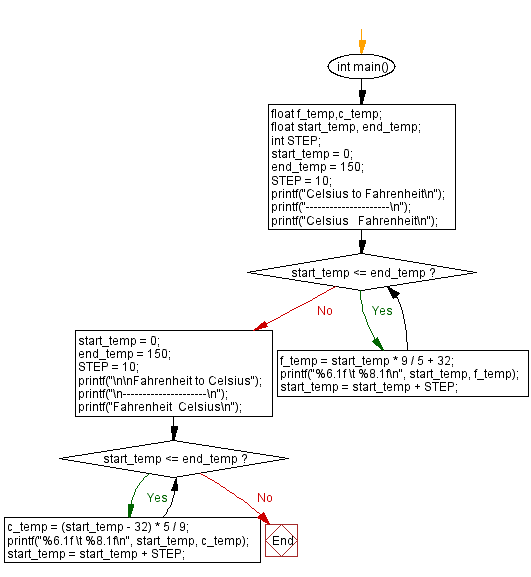﻿ C : Calculate Fahrenheit to Celsius and vice versa

# C Exercises: Print the corresponding Fahrenheit to Celsius and Celsius to Fahrenheit

## C Basic Declarations and Expressions: Exercise-95 with Solution

Write a C program to print the corresponding Fahrenheit to Celsius and Celsius to Fahrenheit.
Both cases initial tempratue = 00, maximum temperature = 1500 and step 100.

Sample Solution:

C Code:

``````#include <stdio.h>

int main() {
float f_temp, c_temp; // Variables to store Fahrenheit and Celsius temperatures
float start_temp, end_temp; // Starting and ending temperatures for the conversion
int STEP; // Step size for temperature increments

// Initialize temperature conversion settings
start_temp = 0;
end_temp = 150;
STEP = 10;

// Fahrenheit to Celsius conversion
printf("Fahrenheit to Celsius");
printf("\n---------------------\n");
printf("Fahrenheit  Celsius\n");

// Loop through the range of Fahrenheit temperatures
while (start_temp<= end_temp) {
// Convert Fahrenheit to Celsius
f_temp = start_temp * 9 / 5 + 32;

// Print the conversion results
printf("%6.1f \t %8.1f\n", start_temp, f_temp);

// Increment the temperature by the specified step size
start_temp = start_temp + STEP;
}

// Reset temperature conversion settings for the second conversion
start_temp = 0;
end_temp = 150;
STEP = 10;

// Celsius to Fahrenheit conversion
printf("\n\nCelsius to Fahrenheit\n");
printf("---------------------\n");
printf("Celsius   Fahrenheit\n");

// Loop through the range of Celsius temperatures
while (start_temp<= end_temp) {
// Convert Celsius to Fahrenheit
c_temp = (start_temp - 32) * 5 / 9;

// Print the conversion results
printf("%6.1f \t %8.1f\n", start_temp, c_temp);

// Increment the temperature by the specified step size
start_temp = start_temp + STEP;
}
}
``````

Sample Output:

```Celsius to Fahrenheit
---------------------
Celsius   Fahrenheit
0.0 	     32.0
10.0 	     50.0
20.0 	     68.0
30.0 	     86.0
40.0 	    104.0
50.0 	    122.0
60.0 	    140.0
70.0 	    158.0
80.0 	    176.0
90.0 	    194.0
100.0 	    212.0
110.0 	    230.0
120.0 	    248.0
130.0 	    266.0
140.0 	    284.0
150.0 	    302.0

Fahrenheit to Celsius
---------------------
Fahrenheit  Celsius
0.0 	    -17.8
10.0 	    -12.2
20.0 	     -6.7
30.0 	     -1.1
40.0 	      4.4
50.0 	     10.0
60.0 	     15.6
70.0 	     21.1
80.0 	     26.7
90.0 	     32.2
100.0 	     37.8
110.0 	     43.3
120.0 	     48.9
130.0 	     54.4
140.0 	     60.0
150.0 	     65.6
```

Flowchart:C programming Code Editor:

What is the difficulty level of this exercise?

Test your Programming skills with w3resource's quiz.

﻿